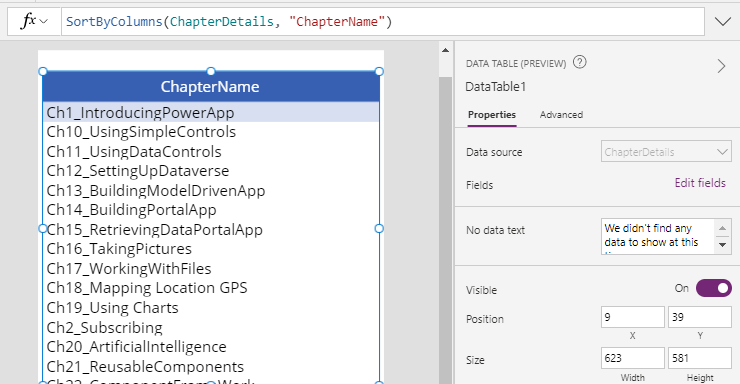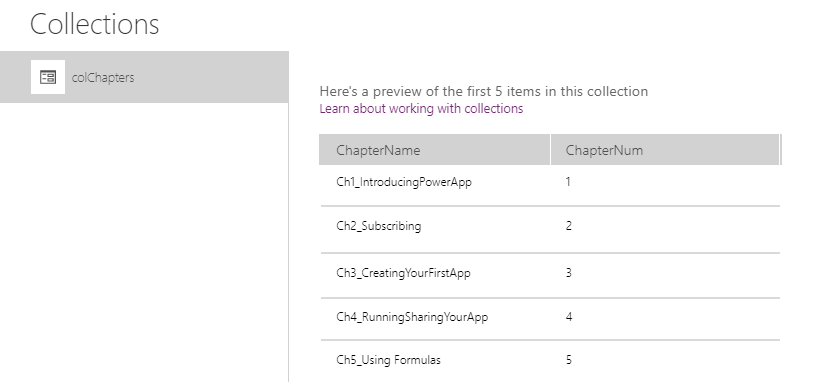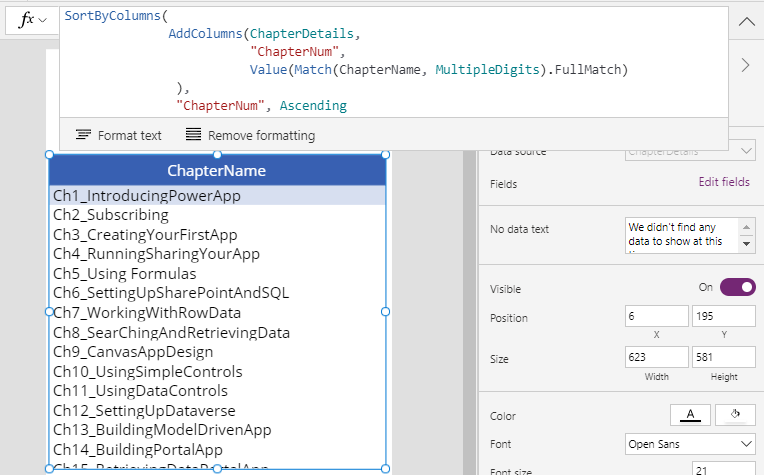# Data - How to sort by partial numbers in a text field

A challenge that we may encounter is, how can we sort a table/list of records by a number that partially appears in a field? An example of where this could occur is where we want to sort manufacturing product descriptions that contain the numeric part number, and we want to sort by the numeric portion of this text. This post describes the formula we can use to carry out this task.

The ability to sort a list of records by a number that appears in a text field can be challenging.

As an example, let's consider the following list of chapter names from an Excel table called ChapterDetails. If we attempt to sort this data by the 'chapter name' column, the output will be sorted by text sequence, and not by the sequence of the number that appears in the chapter name.

The target sort sequence of this data would be "Ch1, Ch2, Ch3....", instead of "Ch1, Ch10, Ch11...".## How to extract the numbers from a text field

To sort this data by the number that appears within the text, it's necessary to extract the number and to sort by the extracted value.

To do this, we can call the Match function to return the digits in the ChapterName field. We can wrap this in a call to AddColumns, to add the output to a column called ChapterNum. Here's how the formula looks.

`AddColumns(ChapterDetails,           "ChapterNum",            Value(Match(ChapterName, MultipleDigits).FullMatch))`

If we were to collect this output to a collection, we would see this result.Note that there is a caveat to this technique in that the Match expression will extract all numbers in the field. Therefore, it would be necessary to incorporate additional text manipulation syntax, if there are numbers present in other parts of the text.

As an example, here is the syntax to extract only the numbers that appear within the first five characters of the chapter name text.

`AddColumns(ChapterDetails,           "ChapterNum",            Value(Match(Left(ChapterName,5), MultipleDigits).FullMatch))`

## Sorting by the extracted number value

Once we build the formula to extract the number into a new column, we can call the Sort/SortByColumn functions to sort the data by the numeric value.

As a demonstration, we can sort the items in a gallery or data table control by setting the Items property to the following formula.

`SortByColumns(              AddColumns(ChapterDetails,                         "ChapterNum",                          Value(Match(ChapterName, MultipleDigits).FullMatch)               ),               "ChapterNum", Ascending)`

The output now appears in the expected sequence, as shown in the screenshot beneath.## Conclusion

To sort a table/list of records by a number that partially appears in a field, we can extract and add the number to a new column by calling the Match and AddColumn functions. We can then sort the records by the numeric value by calling the Sort or SortByColumns function.
•   Categories:
• data